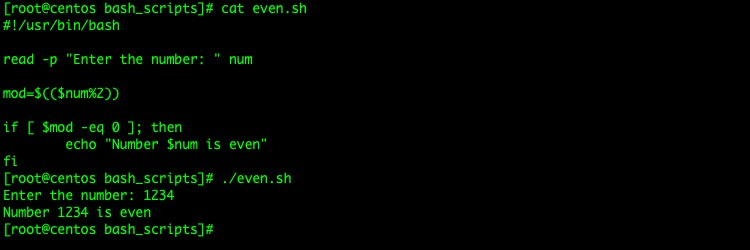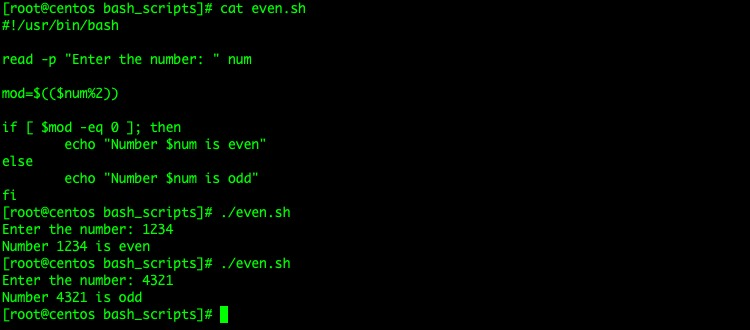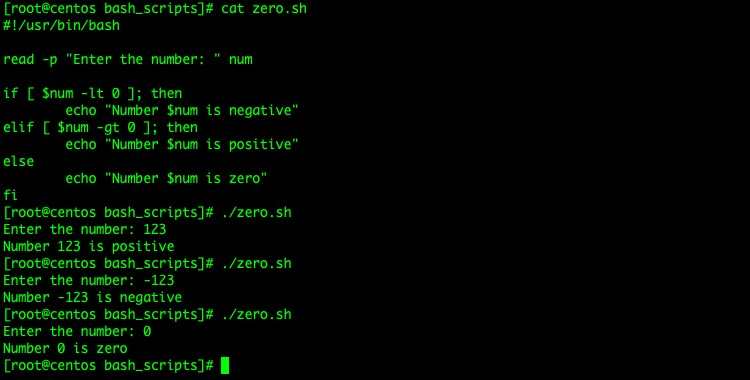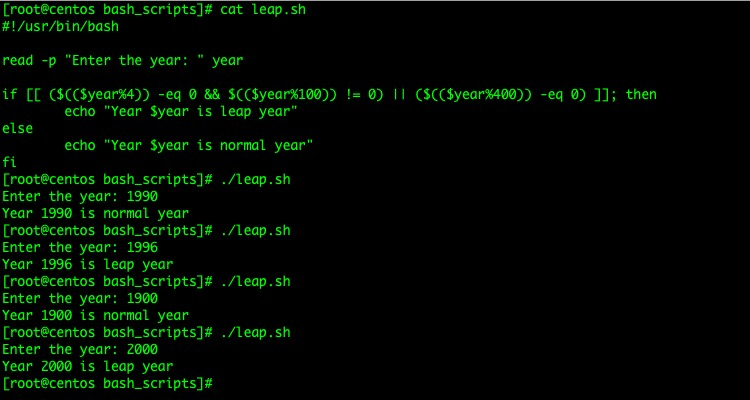Bash 脚本同样支持分支结构，使用 if-else 表达式即可。

```if [ expression ]; then

## execute this block if condition is true else go to next

elif [ expression ]; then

## execute this block if condition is true else go to next

else

## if none of the above conditions are true, execute this block

fi```

• elif 指代的是”else if”，代表不同的条件分支
• 所有的 if else 表达式最后由 fi 结束
• 测试条件需要使用分号”;”和”then”关键字

\$a -lt \$b \$a < \$b (\$a is less than \$b)
\$a -gt \$b \$a >\$b (\$a is greater than \$b)
\$a -le \$b \$a >\$a <= \$b (\$a is less or equal than \$b)
\$a -ge \$b \$a >= \$b (\$a is greater or equal than \$b)
\$a -eq \$b \$a is equal to \$b
\$a -ne \$b \$a is not equal to \$b

“\$a” = “\$b” \$a is same as \$b
“\$a” == “\$b” \$a is same as \$b
“\$a” != “\$b” \$a is different from \$b
-z “\$a” \$a is empty

-f \$a \$a is a file
-d \$a \$a is a directory
-L \$a \$a is a link

Bash 使用 if 表达式

```#!/usr/bin/bash

read -p "Enter the number: " num

mod=\$((\$num%2))

if [ \$mod -eq 0 ]; then
echo "Number \$num is even"
fi``````#!/usr/bin/bash

read -p "Enter the number: " num

mod=\$((\$num%2))

if [ \$mod -eq 0 ]; then
echo "Number \$num is even"
else
echo "Number \$num is odd"
fi``````#!/usr/bin/bash

read -p "Enter the number: " num

if [ \$num -lt 0 ]; then
echo "Number \$num is negative"
elif [ \$num -gt 0 ]; then
echo "Number \$num is positive"
else
echo "Number \$num is zero"
fi``````#!/usr/bin/bash

read -p "Enter the year: " year

if [[ (\$((\$year%4)) -eq 0 && \$((\$year%100)) != 0) || (\$((\$year%400)) -eq 0) ]]; then
echo "Year \$year is leap year"
else
echo "Year \$year is normal year"
fi```